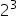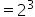Mathematics
Easy

Question

#is called the _____________ form of 8.

## Square    Square root    Cube    IndexHint:

## The correct answer is: Index

### Step 1 of 1:We have to fill the blank in the following sentenceis called the _____________ form of 88 = 2 × 2 × 2Here, 2 is the base and 3 is the index/exponent.So,is the index form of 8.

A base is a number that we will repeatedly multiply to itself

### Related Questions to study#### With Turito Foundation.#### Get an Expert Advice From Turito.